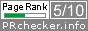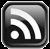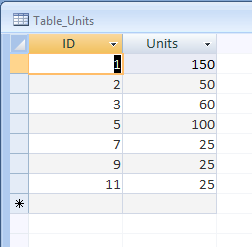# LEARN MS-ACCESS TIPS AND TRICKS

Learn Microsoft Access Advanced Programming Techniques, Tips and Tricks.

### Continuation of earlier Articles:

Hexadecimal Numbers are yet another method to write Binary Values into shorter form than Octal Numbers.  This Numbering System has Number 16 as its Base.  As per the basic rules, which we are following to devise new Numbering Systems, the Base-16 Numbering System have its digit values from 0 to 15 (i.e. one less than the base value 16).  Like decimal numbering system, hexadecimal numbering System also have digit values from 0 to 9. Since, there are no single digit values for 10 to 15 the alphabets A to F are used.

```Hexadecimal Decimal
0            0
1            1
2            2
3            3
4            4
5            5
6            6
7            7
8            8
9            9
A           10
B           11
C           12
D           13
E           14
F           15
```

Now, let us see how we can convert binary numbers into hexadecimal form. We will use the same method we have used for converting Binary to Octal form.  We have made groups of 3 binary digits of binary value 011,111,111 (equal to 255 decimal) to find the Octal Number &O377.  For Hexadecimal Number we must take groups 4 binary digits (Binary 1111 = (1+2+4+8) = 15, the maximum value of a hexadecimal digit) and add the values of binary digits to find the hexadecimal digit.

Let us find the Hexadecimal value of Decimal Number 255.

Decimal 255 = Binary 1111,1111 = Hexadecimal digits FF

It takes 3 digits to write the quantity 255 in decimal as well as in Octal 377 (this may not be true when bigger decimal numbers are converted into Octal) but in Hexadecimal it takes only two digits: FF or ff (not case sensitive).

Let us try another example:

Decimal Number 500 = Binary Number 111110100

111,110,100 =  Octal Number 764

To identify Octal Numbers we have used prefix characters &O or &0 with the Number.  Similarly, Hexadecimal numbers have &H (not case sensitive) as prefix to identify when entered into computers like &H1F4, &hFF etc.

You can type ? &H1F4 in Debug Window of VBA Editing screen to covert and print its Decimal Value.

### MS-Access Functions

There are only two conversion Functions: Hex() and Oct() in Microsoft Access, both uses Decimal Numbers as parameter.

Try the following Examples, by typing them in the Debug Window to convert few Decimal Numbers to Octal and Hexadecimal:

```? OCT(255)
Result: 377

? &O377
Result: 255

? HEX(255)
Result: FF

? &hFF
Result: 255

? HEX(512)
Result: 200

? &H200
Result: 512
```

### MS-Excel Functions

In Microsoft Excel there are Functions for converting values to any of these form.  The list of functions are given below:

1. DEC2BIN()
2. DEC2OCT()
3. DEC2HEX()
4. BIN2DEC()
5. BIN2OCT()
6. BIN2HEX()
7. OCT2DEC()
8. OCT2BIN()
9. OCT2HEX()
10. HEX2DEC()
11. HEX2OCT()
12. HEX2BIN()

You can convert Decimal Value a maximum of 99,999,999 to Octal Number with the Function DEC2OCT() and its equal value of Octal to Decimal OCT2DEC() Function.

499,999,999,999 is the maximum decimal value for DEC2HEX() and its equal value in Hexadecimal form for HEX2DEC() Function.

With the Binary functions you can work with the Decimal value range from 512 to 511 up to a maximum of 10 binary digits (1111111111 the left most bit is the sign bit, i.e. if it is 1 then the value is negative and when 0 positive).

Technorati Tags:
Share:

Comments are subject to moderation before publishing.

## TranslateSubscribe in a readerYour email address:

Delivered by FeedBurner

## Featured Post

### Running Sum in MS-Access Query

Introduction. We are familiar with creating Auto-Numbers in Query Column, through an earlier Function created with the Name QrySeq(), publi...